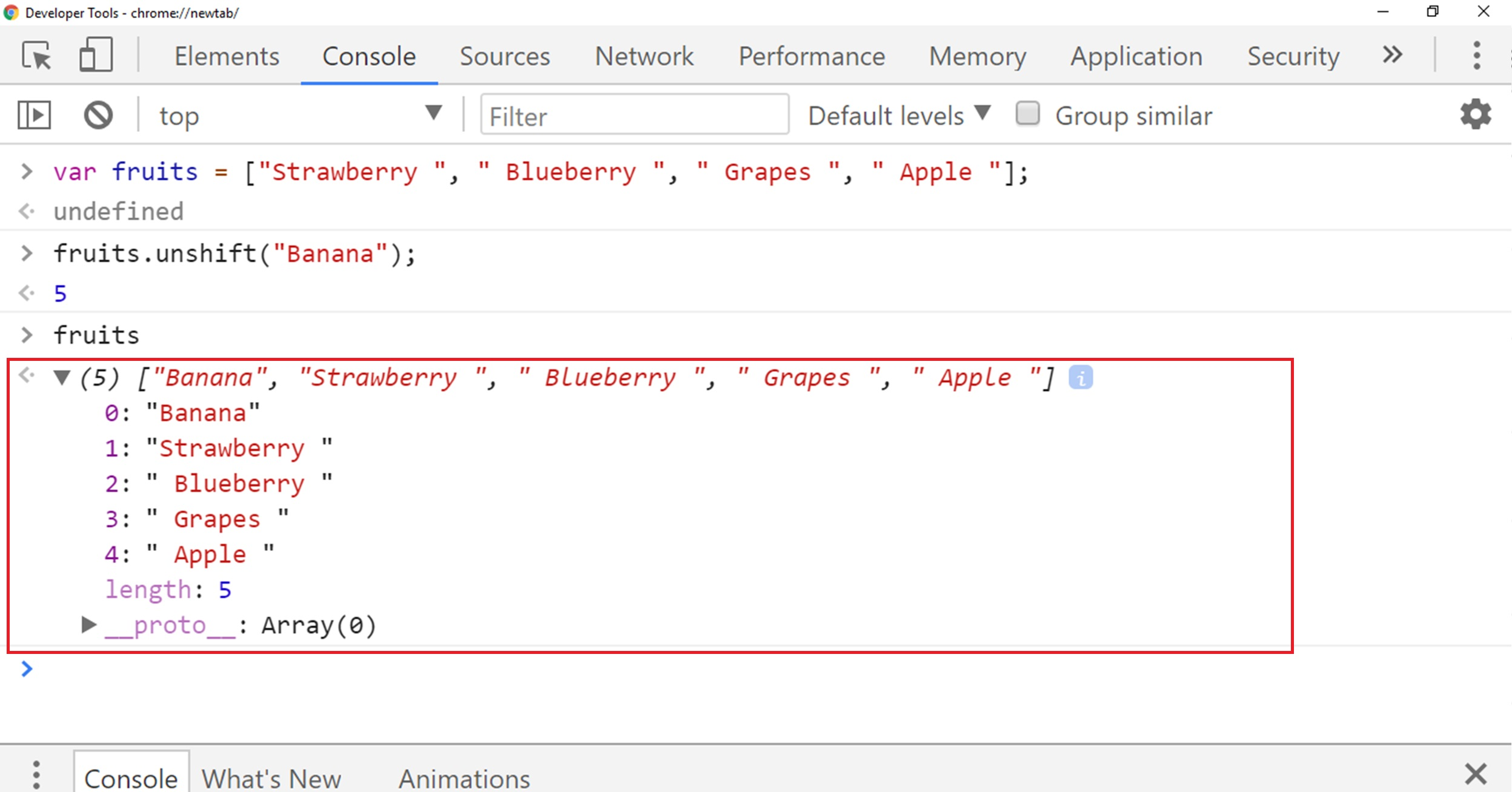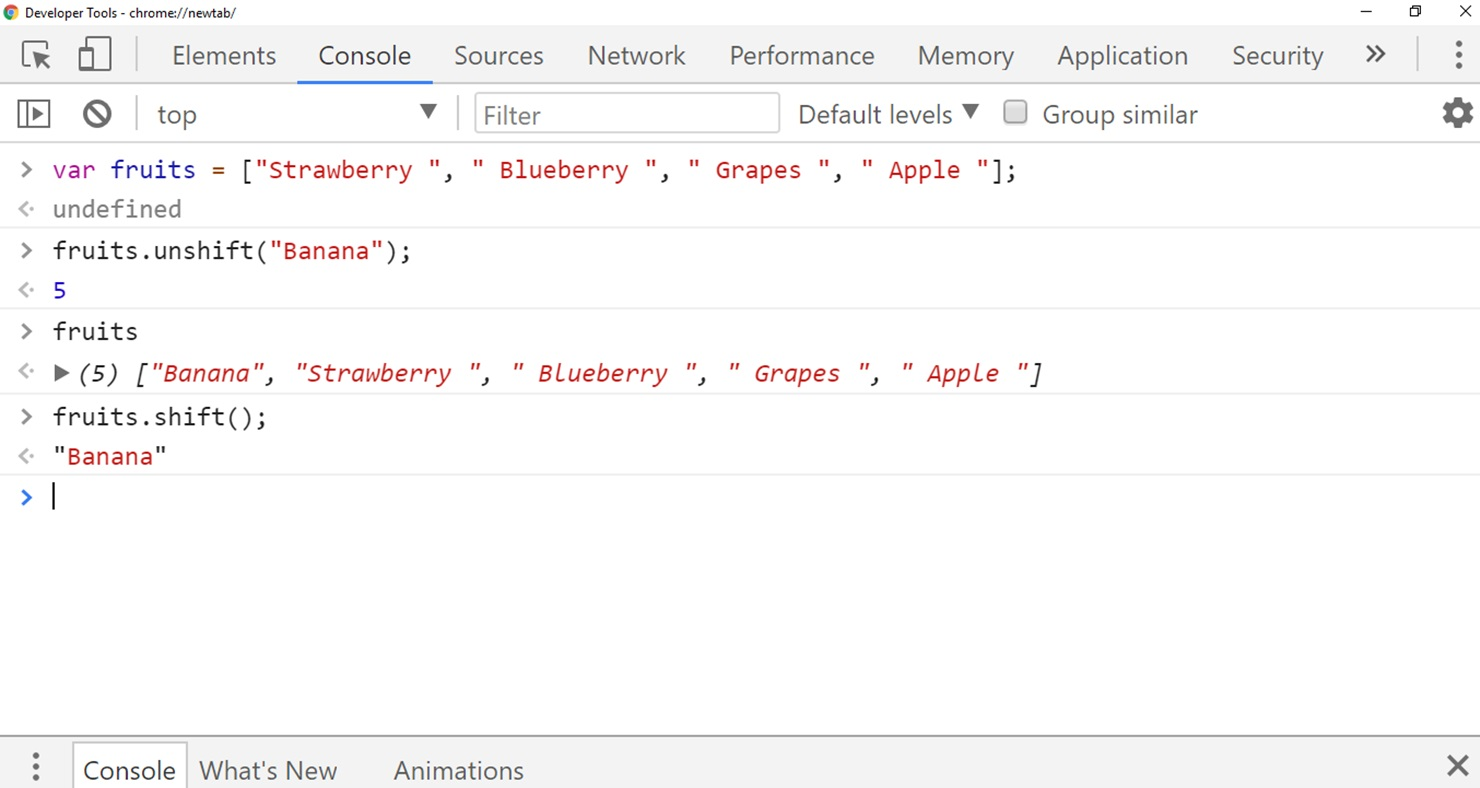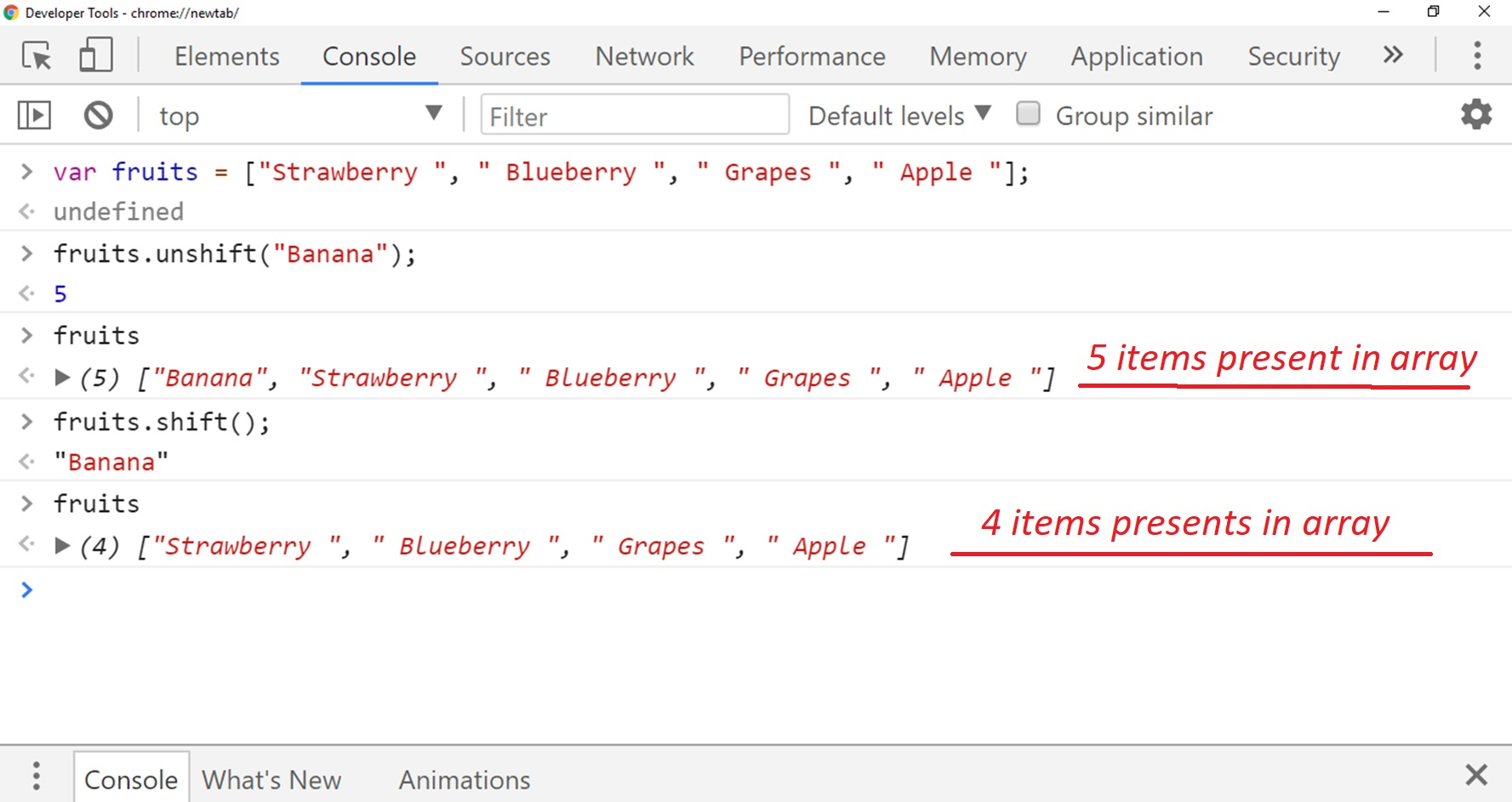# Lesson 18 Shift & Unshift, indexOf and Slice Array Method in Javascript

In this lesson, we are going to discuss more about array method in Javascript, and in this lesson, we will learn about another paired method Shift and Unshift, and learn how we can remove and add items at the beginning of Array list.
We are also going to discuss sort() method there.
So we will also learn how we can arrange array items in alphabetical order.

## Shift and Unshift Method

So shift and unshift is a similar type of array methods that we use in the last lesson that is push and pop, so they work in the very similar way except rather than adding and removing to the end of the array they add and remove from the beginning of the array

## Unshift() Method

Unshift method is much similar to push method, we use shift method to add items at the beginning of an array.
So let’s suppose we have array fruits which consist of four items, here is the code below.

``````var fruits = ["Strawberry ", " Blueberry ", " Grapes ", " Apple "];
``````

## Syntax for Unshift() Method:

If we want to add another item at the beginning of that array we use unshift method.
So let’s suppose If we want to add Banana at the beginning of the list so write:

``````fruits.unshift("Banana");
``````

You can see in the below illustration that how we added another item at the very beginning that is zero index of our array list.In the above illustration, you can notice that we have added 1 element at the beginning of our array list, and returned us the length of the array that is 5.

## Shift() Methods

It’s a built-in JavaScript method, which we use to remove the very first element from the array list.
The syntax is similar to unshift() method but we use shift followed by parentheses instead of unshift().
shift() method does not take any arguments.

## Syntax for Shift() Method:

In our case, we have variable fruit array which consists of 5 items, if we want to remove the 1st item from our array list we use our variable name followed by .Shift() method.
Here is the screenshot of the syntax below:Now we have four elements left in our array list.
So if we want to show our array list we simply type our array name.## Sort() Method

The next array method we are going to discuss there is sort method, so this method we use to sort out our array item and arrange our array item I alphabetical order. So let’s suppose we have
array list:

``````var fruits = ["Strawberry ", " Blueberry ", " Grapes ", " Apple "];
``````

now if I want to arrange this array list in the alphabetical order we will use sort() method, here is the syntax below:

``````fruits.sort();
``````

so in our case, we have fruits arrays, so we use fruits followed by a dot (.) and then sort().
Here is the output below where you can notice how we can arrange an array in alphabetical order.## Conclusion

So in this lesson, we have learned about different types of Array methods and we have learned how we can add or remove the item at the beginning of an array and how we can sort an array in alphabetical order.